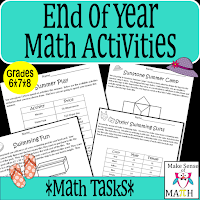# How to Create Higher-Order Thinking Questions for your Math Classroom

Many math teachers know they need to step up the quality of math problems in their classroom, but are not sure how to do it.  I was taught a great strategy and would like to share it with my fellow math teachers.

Teachers are often given math textbooks to teach from, and sadly, open-ended questions are not apart of the majority of math textbooks. However, changing common math problems into open-ended questions is very doable. Here is an idea that I have been taught that has helped to step up the rigor in my math classroom.  Take a common math problem and flip the question and the answer. Students can create questions with the given answer. Take the problem one step further by having them justify their answer either through writing or modeling (or both.)

For example, a common math question might be the following:  "Find the volume of a box with height 3 inches, width 5 inches and length 10 inches."  If you are familiar with Bloom's Taxonomy, I would suggest this falls into the "knowledge" category.  Students simply recall the algorithm and calculate the answer.  While I do believe these questions have their place in a math classroom, they should be in the minority.  What if you instead changed the question to this, "Create a box that has a volume of 150 cubic inches." Suddenly this question now falls into the "synthesis" category, which is considered a higher-order thinking skill.

Here is another example:  Instead of, "Solve the following equation 3x + 2 = 11," flip around the question and say, "Create a two-step equation where the variable equals 3.  Write your equation in two different ways."

Another example: "Find the mean of 12, 15, 18, 20 and 30."  Flip around the question and answer and ask, "What five numbers have a mean of 19 and a range of 18?  Justify your reasoning."

Last example: "Simplify the expressions 2(x + 1) + 4."   Change this problem to "Write three expressions that simplify to 2x + 6.  Prove that your expressions are all equivalent."

Flipping questions does take time, and sometimes it is "easier" to assign the basic problems.  Remember, however, that having students problem-solve and make sense of math will require less review and better retention.  I can assure you that doing so will definitely be worth your time.

If you want a head start on using open-ended questions in your math classroom, here are some great tasks to get started with.  They work great at the end of the year to keep your students engaged, or any time of the year to help your students make sense of math.